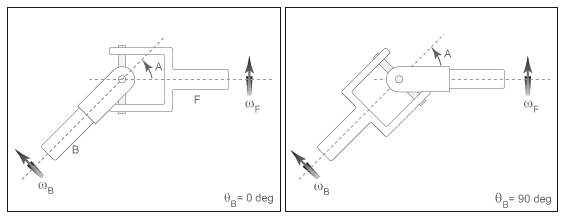# Universal Joint

Rotational coupling between two driveline shafts

• Library:
• Simscape / Driveline / Couplings & Drives

•## Description

The Universal Joint block represents a rotational coupling between two driveline shafts. The coupling transfers torque between the shafts so they spin as a unit under an applied load. Two rotational degrees of freedom, internal to the coupling, allow the shafts to connect at an angle. This intersection angle varies according to the physical signal input from port A. Optional compliance, modeled as a parallel spring-damper set, allows the coupling to deform under load.

You can use the Universal Joint block as a connection between two rotational driveline components—for example, between the driving and driven shafts in an automobile drive train.The ratio of the shaft angular velocities depends on two parameters: the intersection angle between the two shafts and the rotation angle of the base shaft. A physical signal input provides the intersection angle while a property inspector parameter provides the initial base shaft angle. These two angles fix the ratio of the two shaft angular velocities according to the nonlinear equation:

`${\omega }_{F}=\frac{\mathrm{cos}\left(A\right)}{1-{\mathrm{sin}}^{2}\left(A\right)\cdot {\mathrm{cos}}^{2}\left({\theta }_{B}\right)}{\omega }_{B},$`

where:

• ωF is the angular velocity of the follower shaft about its length axis.

• ωB is the angular velocity of the base shaft about its length axis.

• θB is the rotation angle of the base shaft about its length axis.

• A is the intersection angle between base and follower shafts about the base shaft pin.

The two schematics in the figure illustrate the equation parameters. In each schematic, the left shaft represents the base shaft, while the right shaft represents the follower shaft. The right schematic shows the coupling seen in the left schematic after the shafts spin 90° about their length axes (dashed line segments).In the figure, the intersection angle is the angle between the two shafts about the pin of the base shaft. The absolute value of this angle must fall in the range `0 ≤ A < Maximum intersection angle`. The base shaft angle is the angle of the base shaft about its length axis. The base shaft angle is also the time-integral of the base shaft angular velocity, ωB.

## Ports

### Conserving

expand all

Conserving rotational port associated with the base shaft.

Conserving rotational port associated with the folower shaft.

### Input

expand all

Physical signal input port for the intersection angle.

## Parameters

expand all

Maximum intersection angle the joint allows. This angle measures the rotation between base and follower shafts about the base shaft pin. The value of this angle must fall in the range $0\le A rad.

Compliance model for the block:

• `Off` — Do not model stiffness and damping.

• `On` — Model stiffness and damping.

#### Dependencies

If this parameter is set `On`, related parameters are enabled.

Linear spring stiffness of the joint. The spring stiffness accounts for elastic energy storage in the joint due to material compliance.

#### Dependencies

This parameter is enabled when Joint compliance is set to `On`.

Linear damping coefficient of the joint. The damping coefficient accounts for energy dissipation in the joint due to material compliance.

#### Dependencies

This parameter is enabled when Joint compliance is set to `On`.

Rotation angle of the base shaft about its length axis at the beginning of simulation.

Torque that the base shaft transfers to the follower shaft at the beginning of simulation. This torque determines the initial state of material compliance at the joint. Set this value to greater than zero to preload the shafts with torque. Changing this value alters the initial transient response due to material compliance.

#### Dependencies

This parameter is enabled when Jointcompliance is set to `On`.

## Version History

Introduced in R2013b